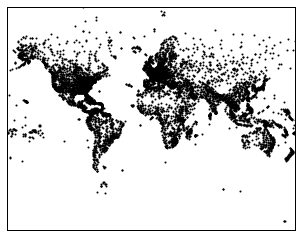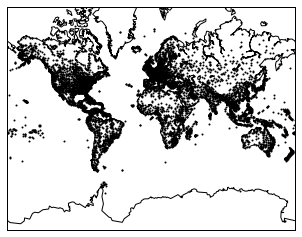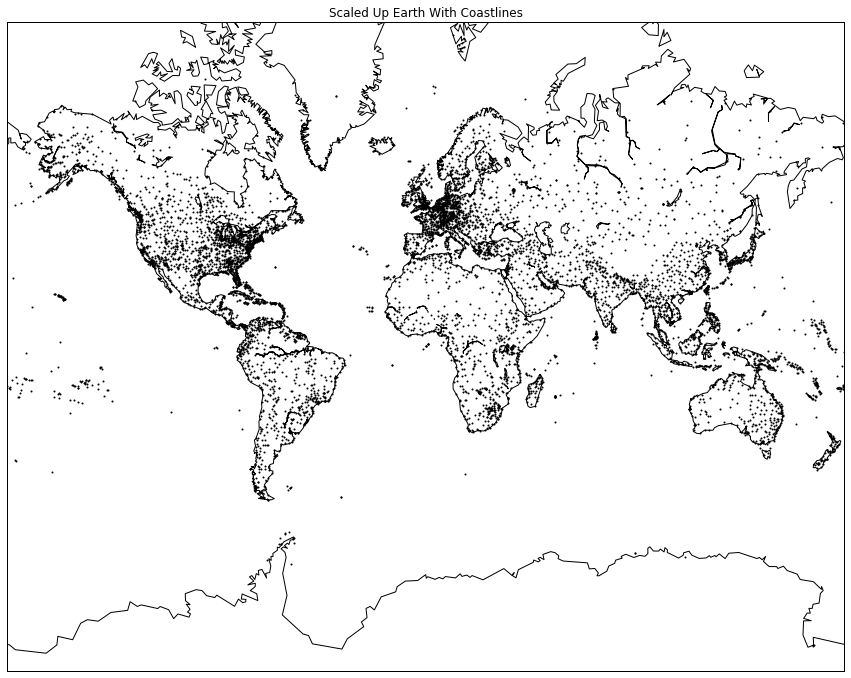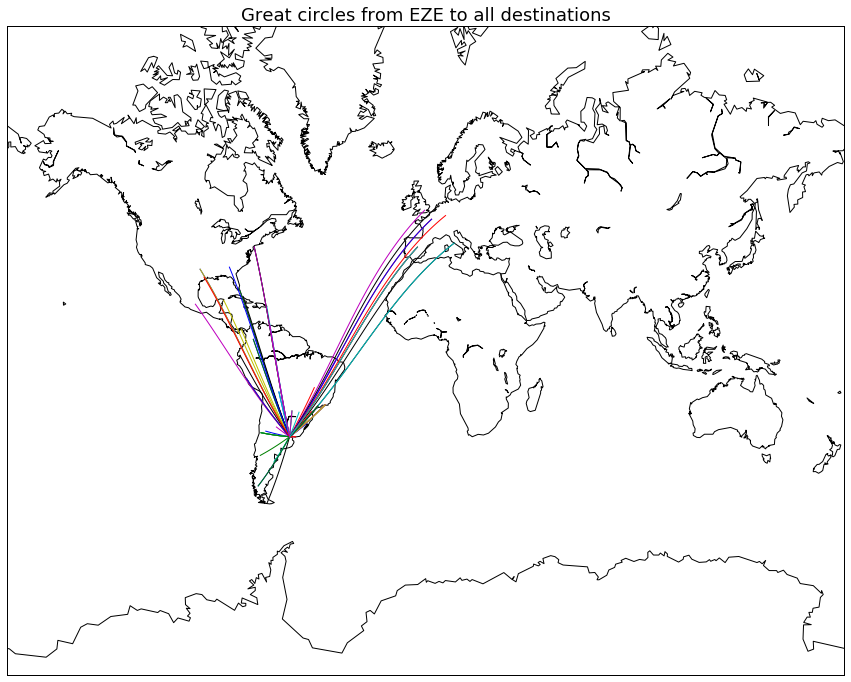# Visualising geographic data with python

This project is part of my learning experience with Dataquest.io where I’m practising using basemap.

## 1. Geographic Data

The datasets were obtained from Openflights.org.

Let’s load pandas and the datasets provided by Dataquest.io:

``````import pandas as pd
``````

With the following commands I can print out the first row of each DataFrame

``````print(airlines.iloc)

print(airports.iloc)

print(routes.iloc)
``````
``````id                       1
name        Private flight
alias                   \N
iata                     -
icao                   NaN
callsign               NaN
country                NaN
active                   Y
Name: 0, dtype: object
id                              1
name                       Goroka
city                       Goroka
country          Papua New Guinea
code                          GKA
icao                         AYGA
latitude                 -6.08169
longitude                 145.392
altitude                     5282
offset                         10
dst                             U
timezone     Pacific/Port_Moresby
Name: 0, dtype: object
airline         2B
airline_id     410
source         AER
source_id     2965
dest           KZN
dest_id       2990
codeshare      NaN
stops            0
equipment      CR2
Name: 0, dtype: object
``````

## 4. Workflow With Basemap

``````import matplotlib.pyplot as plt
from mpl_toolkits.basemap import Basemap

m = Basemap(projection='merc',
llcrnrlat= -80,
urcrnrlat = 80,
llcrnrlon = -180,
urcrnrlon = 180)
``````

You can check out all of the parameters for Basemap. Here’s some comments:

• `projection`: the map projection.
• `llcrnrlat`: latitude of lower left hand corner of the desired map domain
• `urcrnrlat`: latitude of upper right hand corner of the desired map domain
• `llcrnrlon`: longitude of lower left hand corner of the desired map domain
• `urcrnrlon`: longitude of upper right hand corner of the desired map domain

## 5. Converting From Spherical to Cartesian Coordinates

The constructor (`m`) takes only list values, so I can convert longitude and latitude columns from the airports dataframe to lists with `Series.tolist()`.

``````long = airports['longitude'].tolist()
lat = airports['latitude'].tolist()
``````

Now `long` and `lat` lists can be used in a Cartesian coordinate system (since we want the map in 2 dimensions with the Mercador projection). The following will covert the spherical coordinates to Cartesian coordinates.

``````x, y = m(long, lat)
``````

And now I scatter plot the basemap `m`:

``````m.scatter(x, y, s = 1)
plt.show()
``````## 7. Customizing The Plot Using Basemap

We can add costal lines to the map:

``````m = Basemap(projection='merc', llcrnrlat=-80, urcrnrlat=80, llcrnrlon=-180, urcrnrlon=180)
longitudes = airports["longitude"].tolist()
latitudes = airports["latitude"].tolist()
x, y = m(longitudes, latitudes)
m.scatter(x, y, s=1)
m.drawcoastlines()
plt.show()
``````## 8. Customizing The Plot Using Matplotlib

We can add a title and change the size of the figure

``````m = Basemap(projection='merc', llcrnrlat=-80, urcrnrlat=80, llcrnrlon=-180, urcrnrlon=180)
longitudes = airports["longitude"].tolist()
latitudes = airports["latitude"].tolist()
x, y = m(longitudes, latitudes)
fig, ax = plt.subplots(figsize = (15, 20))
ax.set_title('Scaled Up Earth With Coastlines')
m.scatter(x, y, s=1)
m.drawcoastlines()
plt.show()
``````## 9. Introduction to Great Circles

Dataquest.io have another dataset prepared to draw flight routes with great circles. Great circles would be the route of a flight appropriately projected on the 2-dimensional map with the Mercator projection.

``````geo_routes = pd.read_csv('geo_routes.csv')
geo_routes.info()
``````
``````<class 'pandas.core.frame.DataFrame'>
RangeIndex: 67428 entries, 0 to 67427
Data columns (total 8 columns):
airline      67428 non-null object
source       67428 non-null object
dest         67428 non-null object
equipment    67410 non-null object
start_lon    67428 non-null float64
end_lon      67428 non-null float64
start_lat    67428 non-null float64
end_lat      67428 non-null float64
dtypes: float64(4), object(4)
memory usage: 4.1+ MB
airline source dest equipment  start_lon    end_lon  start_lat    end_lat
0      2B    AER  KZN       CR2  39.956589  49.278728  43.449928  55.606186
``````

## 10. Displaying Great Circles

Now the idea is to write a function, named `create_great_circles()` that takes a dataframe and draws a great circle for each route that has an absolute difference in the latitude and longitude values less than 180. And then apply it for a specific airport.

``````fig, ax = plt.subplots(figsize=(15,20))
m = Basemap(projection='merc', llcrnrlat=-80, urcrnrlat=80, llcrnrlon=-180, urcrnrlon=180)
m.drawcoastlines()
``````

This is the function that I wrote

``````def create_great_circles(dataframe):
for row in dataframe.iterrows():
if (row - row < 180) & (abs(row - row) < 180):
m.drawgreatcircle(row, row, row, row)
``````

The iterator iterrows gives a series, so taking `row` selects the actual list of things that I want, since `row` is the dataframe index. Once I have `row` I can select the column that I want – not by its name such as `end_latitude`, but as the column number because now it’s an array and not a series.

My solution above is a bit messier than the function that dataquest.io presented as the answer:

``````def create_great_circles(df):
for index, row in df.iterrows():
end_lat, start_lat = row['end_lat'], row['start_lat']
end_lon, start_lon = row['end_lon'], row['start_lon']

if abs(end_lat - start_lat) < 180:
if abs(end_lon - start_lon) < 180:
m.drawgreatcircle(start_lon, start_lat, end_lon, end_lat)
``````

But anyway, I used my function and chose EZE airport, Ministro Pistarini International Airport in Argentina:

``````dfw = geo_routes[geo_routes['source']=='EZE']
create_great_circles(dfw)
``````And here’s the version with Dublin airport:

``````fig, ax = plt.subplots(figsize = (15, 20))
ax.set_title('Great circles from DUB (Dublin) airport to all destinations', fontsize=18)
m = Basemap(projection='merc', llcrnrlat=-80, urcrnrlat=80, llcrnrlon=-180, urcrnrlon=180)
m.drawcoastlines()

def create_great_circles(dataframe):
for row in dataframe.iterrows():
if (row - row < 180) & (abs(row - row) < 180):
m.drawgreatcircle(row, row, row, row)

dfw = geo_routes[geo_routes['source']=='DUB']
create_great_circles(dfw)
``````